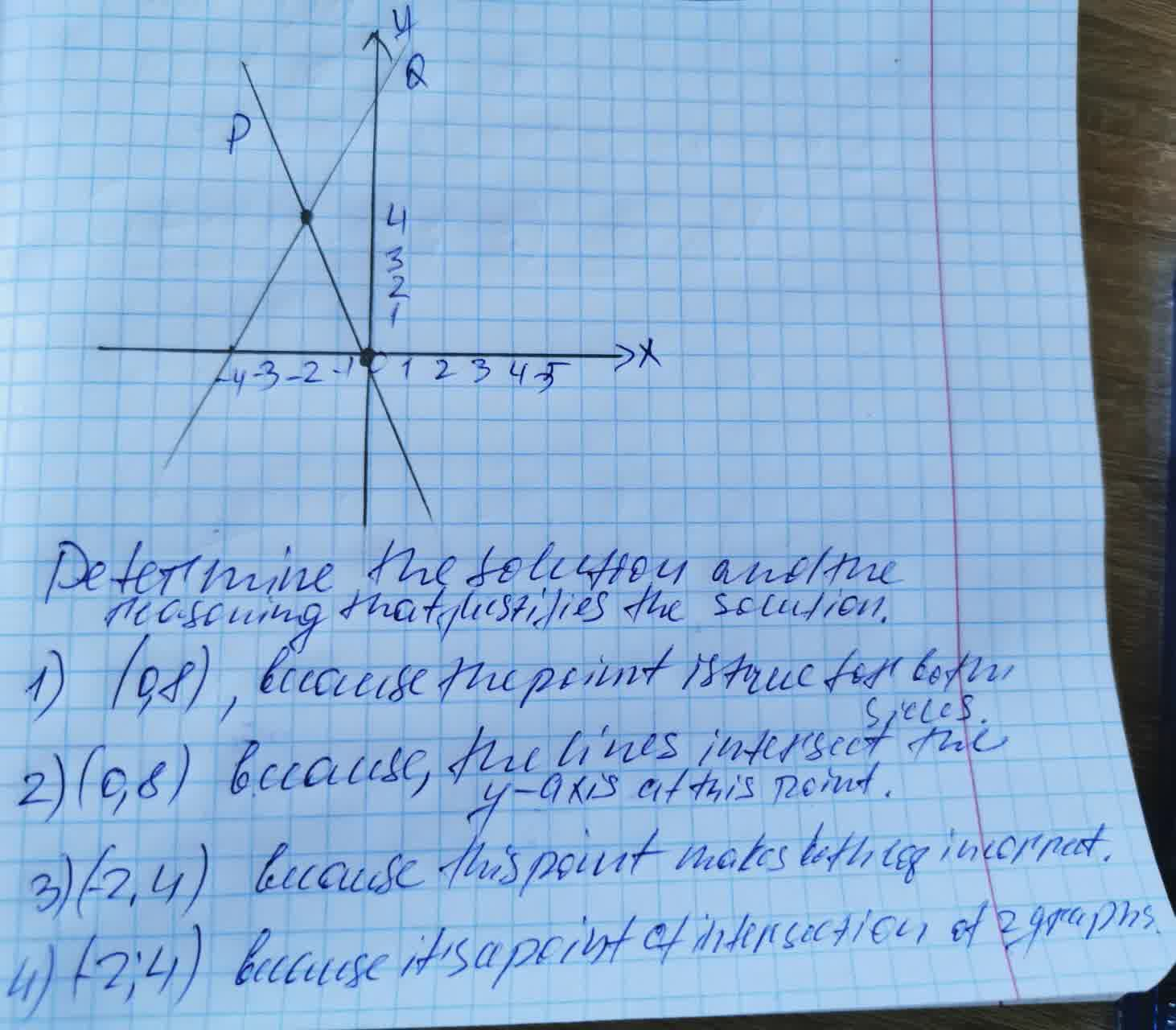# Systems of equations questions and answersRecent questions in Systems of equations
Systems of equations
ANSWERED### Solve the following system of equations: $$5x-4y=-16$$ $$-2x-3y=-12$$

Systems of equations
ANSWERED### Solve the system of equations by hand. $$\begin{cases}-2x+y=5\\ -6x+3y=21\end{cases}$$

Systems of equations
ANSWERED### Consider the system of equations described by $$\begin{cases}x_1=2x_1-3x_2\\x_2=4x_1-5x_2\end{cases}$$ 1. Write down the system of equations in matrix form. 2. Find the eigenvalues of the system of equations. 3. Find the associated eigenvectors.

Systems of equations
ANSWERED### Solve the system of equations by hand. $$\begin{cases}-3x+y=2\\9x-3y=-6\end{cases}$$

Systems of equations
ANSWERED### Solve the system of equations by hand. $$\begin{cases}5x-4y=-5\\3x+y=14\end{cases}$$

Systems of equations
ANSWERED### Solve the system of equations by hand. $$\begin{cases}x+4y=-2\\-2x+12y=9\end{cases}$$

Systems of equations
ANSWERED### Solve the system of equations $$\begin{cases}-7x + 6y =20\\2x -3y=2\end{cases}$$

Systems of equations
ANSWERED### Two lines , P and Q , are graphed:Systems of equations
ANSWERED### x+y=2x+2y=11 3x+3y=62x+4y=22 x+y=2x+2y=11 3x+3y=62x+4y=22 Two systems of equations are shown. Which TWO of the following statements each provide sufficient reasoning to show that the systems have the same solution? The two equations in the second system are multiples of the two equations in the first system. A) The two constant terms in the second system are multiples of the two equations in the first system. B) The graphs of the equations in the first system coincide with the graphs of the equations in the second system. C) The slopes of the graphs of the equations in the first system are equivalent to the slopes of the graphs of the equations in the second system. D) The x -intercepts of the graphs of the equations in the first system coincide with the x -intercepts of the graphs of the equations in the second system. E)

Systems of equations
ANSWERED### Write $$y=13x+7$$ in standard form using integers. a. $$−2x+3y=21$$ b. $$3x−2y=21$$ c. $$−2x−3y=21$$

Systems of equations
ANSWERED### Solve the system of equations $$2x+3y=5$$ $$5x-4y=2$$

Systems of equations
ANSWERED### Solve the system by clennaton $$2x-y=0$$ $$3x-2y=-3$$ The solution is ( . )

Systems of equations
ANSWERED### Substitution and elimination, and matrix methods such as the Gauss-Jordan method and Cramer's rule. Use each method at least once when solving the systems below. include solutions with nonreal complex number components. For systems with infinitely many solutions, write the solution set using an arbitrary variable. $$\displaystyle{y}={x}^{{{2}}}+{6}{x}+{9}$$ $$x+y=3$$

Systems of equations
ANSWERED### Which system of equations is not a linear system? a) $$2x + y = 11$$ $$x = 13 + y$$ b) $$2x = 11 - y$$ $$4x - y = 13$$ c) $$\displaystyle-\frac{{1}}{{2}}{x}-{y}=\frac{{3}}{{4}}$$ $$\displaystyle\frac{{3}}{{2}}{x}+{2}=-\frac{{7}}{{8}}$$ d) $$\displaystyle-{x}²+{y}={10}$$ $$x + y = 5$$

Systems of equations
ANSWERED### Substitution and elimination, and matrix methods such as the Gauss-Jordan method and Cramer's rule. Use each method at least once when solving the systems below. include solutions with nonreal complex number components. For systems with infinitely many solutions, write the solution set using an arbitrary variable. $$x-3y=7$$ $$-3x+4y=-1$$

Systems of equations
ANSWERED### Solve $$\begin{cases}(x-3)^2+(y+1)^2=5\\x-3y=7\end{cases}$$

Systems of equations
ANSWERED### Write and solve a system of equations for each situation. Check your answers A shop has one-pound bags of peanuts for $2 and three-pound bags of peanuts for$5.50. If you buy 5 bags and spend \$17, how many of each size bag did you buy?

Systems of equations
ANSWERED### To find the equation: $$-2y+y=6$$ $$4x-2y=5$$

Systems of equations
ANSWERED### What is the solution of the system of equations? $$y = 2x - 3$$ $$5x + y = 11$$ $$A (2, 1)$$ $$B (1, 2)$$ $$C (3, -4)$$ $$D (1, -1)$$
ANSWERED